# Combination puzzle

Combination puzzle

A combination puzzle, also known as a sequential move puzzle, is a puzzle which consists of a set of pieces which can be manipulated into different combinations by a group of operations. The puzzle is solved by achieving a particular combination starting from a random (scrambled) combination. Often, the solution is required to be some recognisable pattern such as 'all like colours together' or 'all numbers in order'. The most famous of these puzzles is the original Rubik's Cube, a cubic puzzle in which each of the six faces can be independently rotated. Each of the six faces is a different colour, but each of the nine pieces on a face is identical in colour, in the solved condition. In the unsolved condition colours are distributed amongst the pieces of the cube.

The mechanical construction of the puzzle will usually define the rules by which the combination of pieces can be altered. This leads to some limitations on what combinations are possible. For instance, in the case of the Rubiks Cube, there are a large number of combinations that can be achieved by randomly placing the coloured stickers on the cube, but not all of these can be achieved by manipulating the cube rotations. Similarly, not all the combinations that are mechanically possible from a disassembled cube are possible by manipulation of the puzzle. Since neither unpeeling the stickers nor disassembling the cube is an allowed operation, the possible operations of rotating various faces limit what can be achieved.

Although a mechanical realization of the puzzle is usual, it is not actually necessary. It is only necessary that the rules for the operations are defined. The puzzle can be realized entirely in virtual space or as a set of mathematical statements. In fact, there are some puzzles that can only be realized in virtual space. An example is the 4-dimensional 3×3×3×3 tesseract puzzle, simulated by the MagicCube4D software.

## Properties

There have been many different shapes of Rubik type puzzles constructed. As well as cubes, all of the regular polyhedra and many of the semi-regular and stellated polyhedra have been made.

### Regular cuboids

A cuboid is a rectilinear polyhedron. That is, all its edges form right angles. Or in other words (in the majority of cases), a box shape. A regular cuboid, in the context of this article, is a cuboid puzzle where all the pieces are the same size in edge length.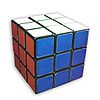Commercial name: Rubik's Cube
Geometric shape: Cube
Piece configuration: 3×3×3

The original Rubik's Cube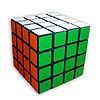Commercial name: Rubik's Revenge
Geometric shape: Cube
Piece configuration: 4×4×4

Solution is much the same as 3×3×3 cube except additional (and relatively simple) algorithm(s) are required to unscramble the centre pieces and edges and additional parity not seen on the 3x3x3 Rubik's Cube.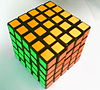Commercial name: Professor's Cube
Geometric shape: Cube
Piece configuration: 5×5×5

Solution is much the same as 3×3×3 cube except additional (and relatively simple) algorithm(s) are required to unscramble the centre pieces and edges.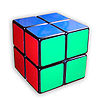Commercial name: Pocket Cube
Geometric shape: Cube
Piece configuration: 2×2×2

Simpler to solve than the standard cube in that only the algorithms for the corner pieces are required. It is nevertheless surprisingly non-trivial to solve.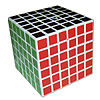Commercial name: V-CUBE
Geometric shape: Cube
Piece configuration: 2×2×2 to 11×11×11
Main articles: V-Cube 6, V-Cube 7

Panagiotis Verdes holds a patent to a method which is said to be able to make cubes up to 11×11×11. He has fully working products for 2×2×2, 5×5×5, 6×6×6 and 7×7×7 cubes. Solution strategies are similar to those of the 4×4×4 and 5×5×5.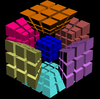4-Dimensional puzzle
Geometric shape: Tesseract
Piece configuration: 3×3×3×3

This is the 4-dimensional analog of a cube and thus cannot actually be constructed. However, it can be drawn or represented by a computer. Seriously more difficult to solve than the standard cube, although the techniques follow much the same principles. There are many other sizes of virtual cuboid puzzles ranging from the trivial 3×3 to the unsolved 5-dimensional 7×7×7×7×7.

Non-uniform cuboids
Geometric shape: Cuboid
Piece configuration (1st): 2×2×3
Piece configuration (2nd): 2×3×3
Piece configuration (3rd): 3×4×4
Piece configuration (4th): 2×2×6

Most of the puzzles in this class of puzzle are generally custom made in small numbers. Most of them start with the internal mechanism of a standard puzzle. Additional cubie pieces are then added, either modified from standard puzzles or made from scratch. The four shown here are only a sample from a very large number of examples. Those with two or three different numbers of even or odd rows also have the ability to change their shape.


Siamese cubes
Geometric shape: Fused cubes
Piece configuration: two 3×3×3 fused 1×1×3

Siamese cubes are two or more puzzles that are fused so that some pieces are common to both cubes. The picture here shows two 3×3×3 cubes that have been fused. The largest example known to exist is in The Puzzle Museum  and consists of three 5×5×5 cubes that are siamese fused 2×2×5 in two places.


Extended cubes
Geometric shape: Box
Piece configuration: 3×3×3 extended 3×3×5

These puzzles are made by bonding additional cubies to an existing puzzle. They therefore do not add to the complexity of the puzzle configuration, they just make it look more complex. Solution strategies remain the same, though a scrambled puzzle can have a strange appearance.


Commercial name: Boob cube
Geometric shape: Box
Piece configuration: 1×1×2

Very possibly the simplest regular cuboid puzzle to solve. Completely trivial solution as the puzzle consists of only two cubies.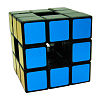Commercial name: Void cube
Geometric shape: Menger Sponge with 1 iteration
Piece configuration: 3x3x3 with a hole in the middle.

Solutions to this cube is similar to a regular 3x3x3. However, parity errors are possible in these solutions. This cube uses a special mechanism due to absence of a central core.

#### Pattern variations

There are many puzzles which are mechanically identical to the regular cuboids listed above but have variations in the pattern and colour of design. Some of these are custom made in very small numbers, sometimes for promotional events. The ones listed in the table below are included because the pattern in some way affects the difficulty of the solution or is notable in some other way.

No Picture

Commercial name: Junior Cube
Geometric shape: Cube
Piece configuration: 2×2×2

Mechanically identical to the Pocket Cube. However, much easier to solve as it only uses two colours.


Commercial name: Fooler Cube
Geometric shape: Cube
Piece configuration: 3×3×3

Mechanically identical to the standard 3×3×3 cube but not a real puzzle since all the faces are the same colour. There are also cubes which have only three colours, either one colour per pair of opposite faces or one colour per layer. Also known as the Dodo cube.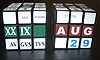Commercial name: Calendar Cube
Geometric shape: Cube
Piece configuration: 3×3×3

Mechanically identical to the standard 3×3×3 cube, but with specially printed stickers for displaying the date. Much easier to solve since five of the six faces are ignored. Ideal produced a commercial version during the initial cube craze. Sticker sets are also available for converting a normal cube into a calendar.


Rubik's Cube for the blind
Geometric shape: Cube
Piece configuration: 3×3×3

Mechanically identical to the standard 3×3×3 cube. However the pieces are in some way tactile to allow operation by blind persons, or to solve blindfolded. The cube pictured is the original "Blind Man's Cube" made by Politechnika. This is coloured the same as the standard cube, but there is an embossed symbol on each square which corresponds to a colour.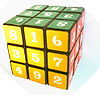Commercial Name: Magic Cube
Geometric shape: Cube
Piece configuration: 3×3×3

Mechanically identical to the standard 3×3×3 cube. However, the numbers on the centre pieces force the solver to become aware that each one can be in one of four orientations, thus hugely increasing the total number of combinations. The number of combinations of centre face orientations is 46. However, odd combinations (overall odd number of rotations) of the centre faces cannot be achieved with legal operations. The increase is therefore x211 over the original making the total approximately 1024 combinations. This adds to the difficulty of the puzzle but not astronomically; only one or two additional algorithms are required to effect a solution. Note that the puzzle can be treated as a number magic square puzzle on each of the six faces with the magic constant being 15 in this case.


Patterned cubes
Geometric shape: Cube
Piece configuration: 3×3×3

Mechanically identical to the standard 3×3×3 cube. The pattern, which is often a promotional logo or pictures of performers, will usually have the effect of making the orientation of the centre pieces 'count' in the solution. The solution is therefore the same as the 'Magic Square' cube above.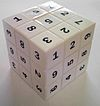Commercial name: Sudoku Cube
Geometric shape: Cube
Piece configuration: 3×3×3

Identical to the Rubik's Cube in mechanical function, it adds another layer of difficulty in that the numbers must all have the same orientation and there are no colors to follow. The name reflects its superficial resemblance to the two-dimensional Sudoku number puzzle.
Over The Top

Commercial name: Over The Top
Geometric shape: Cube
Piece configuration: 17x17x17
Inventor: Oskar van Deventer

A remarkable extension to the basic Rubik's Cube. Experimental; made by 3-D printing of plastic. Corners are much larger in proportion, and edge pieces match that larger dimension; they are narrow, and do not resemble cubes. The rest of the cubelets are 15x15 arrays on each side of the whole cube; as planned, they would be only 4 mm on a side. The mechanism is a 3x3x3 core, with thin "vanes" for the center edges; the rest of the cubelets fill in the gaps. The core has a sphere at its center.

Surrounding the core are six concentric spherical shells (or more, depending on your definition). The scheme is quite different from that of Panagiotis Verdes, the inventor of the V Cubes. Mr. van Deventer is a noted inventor of puzzles.

### Irregular cuboids

An irregular cuboid, in the context of this article, is a cuboid puzzle where not all the pieces are the same size in edge length. This category of puzzle is often made by taking a larger regular cuboid puzzle and fusing together some of the pieces to make larger pieces. In the formulae for piece configuration, the configuration of the fused pieces is given in brackets. Thus, (as a simple regular cuboid example) a 2(2,2)x2(2,2)x2(2,2) is a 2×2×2 puzzle, but it was made by fusing a 4×4×4 puzzle. Puzzles which are constructed in this way are often called "bandaged" cubes. However, there are many irregular cuboids that have not (and often could not) be made by bandaging.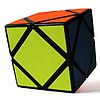Commercial name: Skewb
Geometric shape: Cube
Piece configuration: -

Similar to the original Rubik's Cube, the Skewb differs in that its four axes of rotation pass through the corners of the cube rather than the centres of the faces. As a result, it is a deep-cut puzzle in which each twist scrambles all six faces.


Bandaged Cubes
Geometric shape: Cube
Piece configuration: various

The example shown in the link is a simple example of a large number of bandaged cubes that have been made.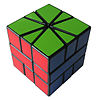Commercial name: Square One
Geometric shape: Cube

A variation on the original Rubik's Cube where it can be turned in such a manner as to distort the cubical shape of the puzzle. The Square One consists of three layers. The upper and lower layers contain kite and triangular pieces. The middle layer contains two trapezoid pieces, which together may form an irregular hexagon or a square. Square One is an example of another very large class of puzzle — cuboid puzzles which have cubies that are not themselves all cuboid.

### Other polyhedra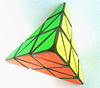Commercial Name: Pyraminx
Geometric shape: Tetrahedron
Piece configuration: 3×3×3

Pyramid shaped puzzle similar to Rubik's cube in operation and solution.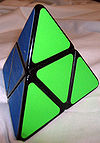Commercial Name: Pyramorphix
Geometric shape: Tetrahedron
Piece configuration: 2×2×2

Pyramid shaped puzzle with a 2×2×2 cube mechanism.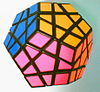Commercial Name: Megaminx
Geometric shape: Dodecahedron
Piece configuration: Similar to 3×3×3

12-sided polyhedron puzzle similar to Rubik's cube in operation and solution.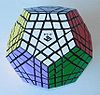Commercial Name: Gigaminx, Teraminx, Petaminx
Geometric shape: Dodecahedron

Megaminx variants with multiple layers per face. The Gigaminx has 2 layers per face, for a total of 5 layers per edge; the Teraminx has 3 layers per face, 7 layers per edge; and the Petaminx has 4 layers per face, 9 layers per edge.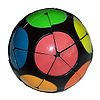Commercial Name: Impossiball
Geometric shape: Rounded icosahedron
Piece configuration: -

Rounded icosahedron puzzle similar to Pocket Cube in operation and solution.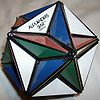Commercial Name: Alexander's Star
Geometric shape: Great dodecahedron
Piece configuration: -

12-sided Nonconvex uniform polyhedron puzzle similar to Rubik's cube in operation and solution.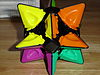Commercial Name: BrainTwist
Geometric shape: Tetrahedron
Piece configuration: -

The BrainTwist is a unique tetrahedral puzzle with an ability to "flip", showing only half of the puzzle at a time.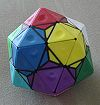Commercial Name: Dogic
Geometric shape: Icosahedron
Piece configuration: -

The Dogic is an icosahedron cut into 60 triangular pieces around its 12 tips and 20 face centers.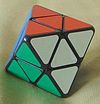Commercial Name: Skewb Diamond
Geometric shape: Octahedron
Piece configuration: 3×3×3

An octahedral variation on the Skewb, it is a deep-cut puzzle very similar to the Skewb and is a dual-polyhedron transformation.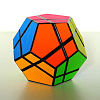Commercial Name: Skewb Ultimate
Geometric shape: Dodecahedron
Piece configuration: -

While appearing more difficult than the Skewb Diamond, it is functionally the same as the Skewb and Skewb Diamond. The puzzle is cut in a different manner but the same solutions can be used to solve it by identifying what pieces are equivalent.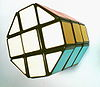Commercial Name: Barrel Cube
Geometric shape: Octagonal Prism
Piece configuration: 3×3×3

Mechanically identical to the 3×3×3 cube. It does, however, have an interesting difference in its solution. The vertical corner columns are different colours to the faces and do not match the colours of the vertical face columns. The corner columns can therefore be placed in any corner. On the face of it, this makes the solution easier, however odd combinations of corner columns cannot be achieved by legal moves. The solver may unwittingly attempt an odd combination solution, but will not be aware of this until the last few pieces.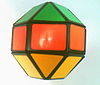Commercial Name: Diamond Cube
Geometric shape: Rhombicuboctahedron
Piece configuration: 3×3×3

Mechanically identical to the 3×3×3 cube although the example pictured is easier to solve due to the restricted colour scheme. This puzzle is a rhombicuboctahedron but not a uniform one as the edge pieces are oblong rather than square. There is in existence a similar puzzle actually called Rhombicuboctahedron which is uniform.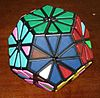Commercial Name: Pyraminx Crystal
Geometric shape: Dodecahedron
Piece configuration: -

A dodecahedron cut into 20 corner pieces and 40 edge pieces. It is similar to the Megaminx, but is deeper cut, giving edges that behave differently from the Megaminx's edges when twisted.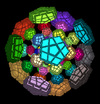Commercial Name: Magic 120-cell
Geometric shape: 120-cell
Piece configuration: 3×3×3×3

Virtual 4-dimensional puzzle, the 4-D analogue of the Megaminx.

### Other geometric shapes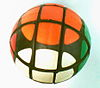Commercial Name: Magic Ball
Geometric shape: Sphere
Piece configuration: 3×3×3

Also known as Rubik's Sphere. Mechanically identical to the 3×3×3 cube in operation and solution. The only practical difference is that it is rather hard to grip. This accounts for the poor condition of this specimen, as the coloured stickers tend to get forced off in use.

### Non-Rubik style three-dimensional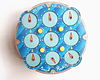Commercial Name: Rubik's Clock
Piece configuration: 3×3×2 12-position dials

Rubik's Clock is a two-sided puzzle, each side presenting nine clocks to the puzzler. There are four wheels, one at each corner of the puzzle, each allowing the corresponding corner clock to be rotated directly.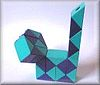Commercial Name: Rubik's Snake
Piece configuration: 24 pieces

Some would not count this as a combinational puzzle despite it bearing the Rubik name. Also known as Rubik's Twist. There is no one solution to this puzzle but multiple different shapes can be made.

### Two-dimensional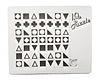Sliding piece puzzle
Piece configuration: 7×7

These ubiquitous puzzles come in many sizes and designs. The traditional design is with numbers and the solution forms a magic square. There have been many different designs, the example shown here uses graphic symbols instead of numbers. The solution requires that there are no repeated symbols in any row column or diagonal. The picture shows the puzzle unsolved.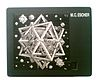Sliding piece puzzle with picture
Piece configuration: 7×7

Mechanically, no different from the puzzle above. However, the picture on the pieces gives the puzzle something of the nature of a jigsaw in addition to being a combination puzzle. Note that the picture consists of multitude of a polyhedra which have been made into Rubik puzzles.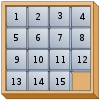Fifteen puzzle
Piece configuration: 4×4-1

The original sliding piece puzzle.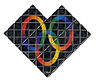Rubik's Magic
Not entirely 2D. Involves flipping parts back onto itself.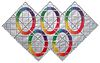Rubik's Master Magic
The five ringed version of the Rubik's Magic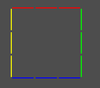Commercial name:2D Magic Cube
Geometric shape:Square
Piece configuration: 3×3

Another virtual puzzle in the Rubik series, but this time a very simple one.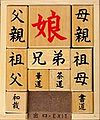Klotski
Piece configuration: 4×5-2 with some fused pieces

A traditional sliding piece puzzle. There are now endless variations of this original puzzle implemented as computer games.

Wikimedia Foundation. 2010.

### Look at other dictionaries:

• Combination puzzles — Combination puzzles, also called sequential move puzzles, consist of a set of pieces which can be manipulated into different combinations by a group of operations. The puzzle consists of achieving a particular combination starting from a random… …   Wikipedia

• Puzzle — For other uses, see Puzzle (disambiguation). Puzzle solving redirects here. For the concept in Thomas Kuhn s philosophy of science, see normal science. Part of a series on Puzzles …   Wikipedia

• Puzzle video game — Part of a series on Puzzles …   Wikipedia

• puzzle lock — noun : combination lock …   Useful english dictionary

• Mechanical puzzle — Part of a series on Puzzles …   Wikipedia

• Square One (puzzle) — This article is about the puzzle. For the popular phrase, see Back to square one. The Square One, also known as Back to Square One and Cube 21, is a puzzle similar to the Rubik s Cube. Its distinguishing feature among the numerous Rubik s Cube… …   Wikipedia

• List of puzzle video games — Part of a series on Puzzles …   Wikipedia

• Logic puzzle — Part of a series on Puzzles …   Wikipedia

• Disentanglement puzzle — Part of a series on Puzzles …   Wikipedia

• Dissection puzzle — Part of a series on Puzzles …   Wikipedia